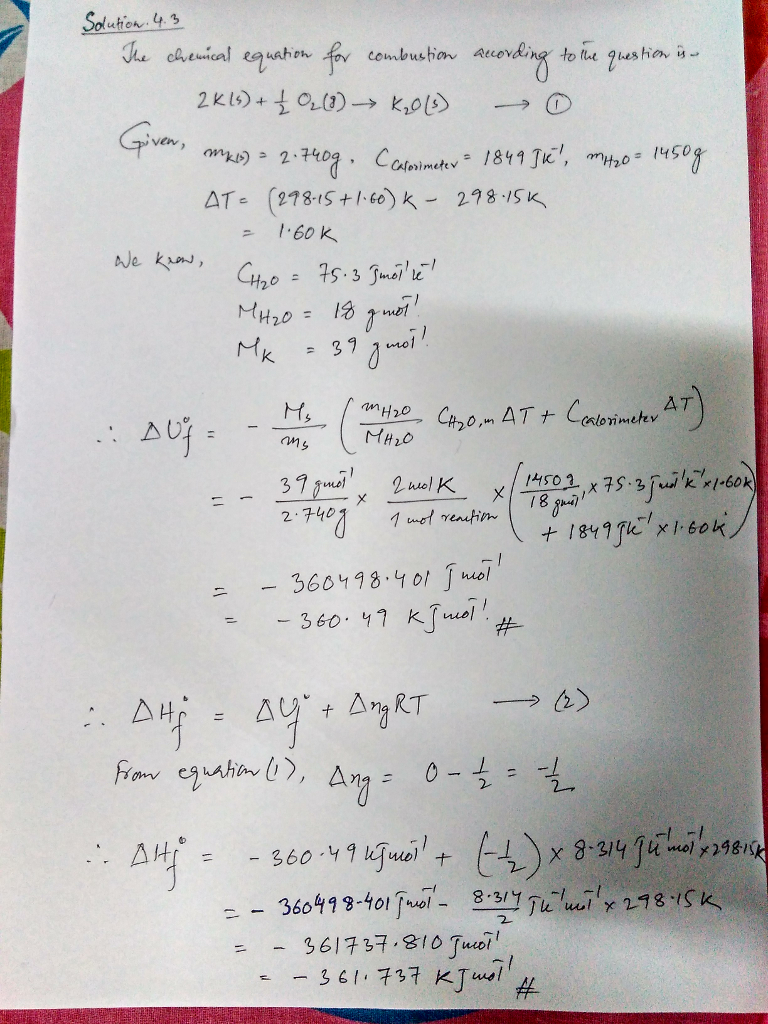# Homework Solution: At 1000 . K, Delta H degree _R = -123.77 kJ mol^-1 for the reaction N_2(g) + 3…

At 1000 . K, Delta H degree _R = -123.77 kJ mol^-1 for the reaction N_2(g) + 3 H_2(g) rightarrow 2 NH_3(g), with C_P,m = 3.502R, 3.466R, and 4.217R for N_2(g), H_2(g), and NH_3(g), respectively. Calculate Delta H degree _R of NH_3(g) at 450. K from this information. Assume that the heat capacities are independent of temperature. A sample of K(s) of mass 2.740 g undergoes combustion in a constant volume calorimeter at 298.15 K. The calorimeter constant is 1849 J K^-1, and the measured temperature rise in the inner water bath containing 1450. g of water is 1.60 K. Calculate Delta U degree _f and Delta H degree _f for K_2O. Calculate Delta H degree _f for NO(g) at 975 K, assuming that the heat capacities of reactants and products are constant over the temperature interval at their values at 298.15 K. The total surface area of the earth covered by ocean is